Lebesgue dimension

(diff) ← Older revision | Latest revision (diff) | Newer revision → (diff)
A dimension defined by means of coverings (cf. Covering (of a set)). It is the most important dimension invariant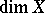of a topological spaceand was discovered by H. Lebesgue . He stated the conjecture that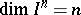for the-dimensional cube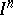. L.E.J. Brouwer  was the first to prove this, as well as the stronger identity:. A precise definition of the invariant(for the class of metric compacta) was given by P.S. Urysohn, who proved that for a spaceof this class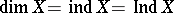For compactathe Lebesgue dimension is defined as the smallest integerhaving the property that for anythere is a finite open-covering ofthat has multiplicity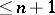; an-covering of a metric space is a covering all elements of which have diameter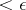, and the multiplicity of a finite covering ofis the largest integersuch that there is a point ofcontained inelements of the given covering. For an arbitrary normal (in particular, metrizable) spacethe Lebesgue dimension is the smallest integersuch that for any finite open coveringofthere is a (finite open) coveringof multiplicitythat refines it. A coveringis said to be a refinement of a coveringif every element ofis a subset of at least one element of.